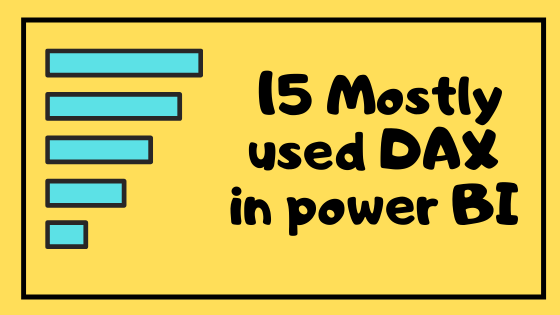# Mostly used 15 DAX functions in power BI## Introduction

Data Analysis Expressions (DAX) is used to compute the data in the power BI. DAX can be used to create measure as well as calculated column. It is not a programming language; it is a formula as well as query language. If you unleash the power of DAX, then you can become a power BI expert.

In this article, we are going to see the 15 DAX functions mostly used by powerbi developers with its syntax.

## What is Measure?

Measure is an aggregated output value created in power BI by using different pre-defined functions as well as user defined functions. It will be calculated dynamically based on the column used with it.

## What is expression?

A sequence of operands and operators is called an expression.

Eg:  [unit price] * [quantity] – [discount]. Here unit price, Quantity and discount are operands and *,- are operator.

## Content:

DAX can be used to create measure as well as calculated column. In here among 15 DAX first 8 will be measures and the remaining 7 are calculated column/table.

1. SUM
2. AVERAGE
3. MIN
4. MAX
5. CALCULATE
6. COUNT/DISTINCTCOUNT
7. COUNTROWS
8. DISTINCT
9. FILTER
10. PREVIOUSDAY
11. DATEDIFF
12. CALENDAR
13. RELATED
14. TOTALYTD
15. CALCULATETABLE

### SUM

The sum function adds the value in the column of a table. The syntax is given below:

SUM (column name)

### AVERAGE

The average function gives the average value of a column from the table. The syntax is given below:

AVERAGE (column name)

### MIN

The Min function finds the minimum value in a column or minimum value between two expressions. The syntax is given below.

MIN(columnname), MIN(expression1, expression2)

### MAX

The Max function finds the maximum value in a column or maximum value between two expressions. The syntax is given below.

MAX(columnname), MAX(expression1, expression2)

### CALCULATE

The calculate function used to compute complex functions with the conditions mentioned in the filter variable. User can add as many filter as they need. The syntax is given below.                          CALCULATE(expression, filter1,filter2,…)

### COUNT

The count function used to count the number of values in a column. Null values are not included The syntax is given below:

COUNT(column name)

### COUNTROWS

The count rows function returns the number of rows in the given table. The syntax is given below:

COUNTROWS(table name)

### DISTINCT

1.      The function distinct can be used on column as well as table. It will return a one column distinct table or distinct row table. The syntax for count function is given below:

DISTINCT(column name), DISTINCT(table name)

### FILTER

The count function used to count the number of values in a column. Null values are not included The syntax is given below:

COUNT(column name)

### PREVIOUSDAY

This function returns a column that contains previous day of each value. The syntax is given below:

PREVIOUSDAY(date column)

### DATEDIFF

This function returns the date difference in number based on the interval given. The syntax is given below:

DATEDIFF(start date, end date, interval)

### CALENDAR

This function returns the one column table with date as column name. The range of dates is taken from start date and end date. The syntax is given below:

CALENDAR(start date, end date)

### RELATED

This function returns related column value from another table based on the relationship mentioned between the tables. The syntax is given below:

RELATED(column name)

### TOTALYTD

This function returns the aggregated output based on the dates passed and the output is filtered on current year to today’s date. The syntax is given below:

TOTALYTD(expression, dates)

### CALCULATETABLE

This function returns table of values with filters applied on it. The syntax is given below:

CALCULATETABLE( expression,filter1,filter2,…)

Most of the functions have been used in the sample power BI report provided in the Microsoft website. Check it out using the below link. click here

## Author

•Tableau Certified Data Analytics professional with 7+ years of overall IT experience and 4 years of experience in analytics. Completed Post graduate program in Data science and Engineering from Great Lakes. Having good knowledge in Tableau, PowerBI, SQL and Python. Member of Data Visualization Society(DVS). Currently working as Data Analyst in Elsevier, a publishing and information analytics company.